# Computing the Bend AllowanceThis section explains the calculations related to folding/unfolding operations.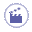Click SheetMetal Parameters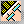.

 The Sheet Metal Parameters dialog box is displayed. The third tab concerns the bend allowance.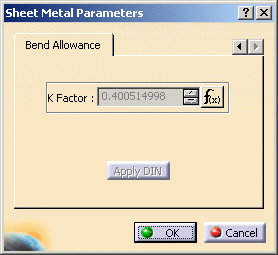• Bend Allowance
The bend allowance corresponds to the unfolded bend width.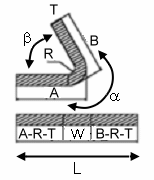bend < 90deg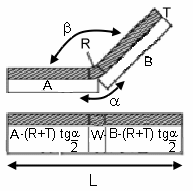bend > 90deg

L is the total unfolded length
A and B the dimensioning lengths as defined on the above figure. They are similar to the DIN definition.

• K Factor
Physically, the neutral fiber represents the limit between the material compressed area inside the bend and the extended area outside the bend. Ideally, it is represented by an arc located inside the thickness and centered on the bend axis.
The K factor defines the neutral fiber position:
W = a * (R + k * T)

where:

W is the bend allowance
R the inner bend radius
T the sheet metal thickness
a the inner bend angle in radians.

If b is the opening bend angle in degrees:

a = p * (180 - b) / 180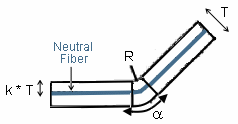When you define the sheet metal parameters, a literal feature defines the default K Factor and a formula is applied to implement the DIN standard. This standard is defined for thin steel parts. Therefore the K Factor value ranges between 0 and 0.5.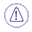The DIN definition for the K factor slightly differs.
W = a * (R + k' * T/2)
Therefore k' = 2 * k and ranges from 0 to 1.
This formula can be deactivated or modified by right-clicking in the K factor field and choosing an option from the contextual menu. It can be re-activated by clicking the Apply DIN button. Moreover, the limit values can also be modified.
When a bend is created, its own K Factor literal is created.
Two cases may then occur:
1. If the Sheet Metal K Factor has an activated formula using the default bend radius as input parameter, the same formula is activated on the bend K Factor replacing the default bend radius by the local bend radius as input.
2. In all other cases, a formula "equal to the Sheet Metal K Factor" is activated on the local bend K Factor.
This formula can also be deactivated or modified.

• Bend Deduction
When the bend is unfolded, the sheet metal deformation is thus represented by the bend deduction V, defined by the formula:
L = A + B + V
(refer to the previous definitions).

Therefore the bend deduction is related to the K factor using the following formula:

V = a * (R + k * T) - 2 * (R + T) * tan ( min(p/2,a) / 2)
This formula is used by default. However, it is possible to define bend tables on the sheet metal parameters. These tables define samples: thickness, bend radius, open angle, and bend deduction. In this case, the bend deduction is located in the appropriate bend table, matching thickness, bend radius, and open angle. If no accurate open angle is found, an interpolation will be performed.
When updating the bend, the bend deduction is first computed using the previously defined rules. Then the bend allowance is deduced using the following formula:

W = V + 2 * (R + T) * tan ( min(p/2,a) / 2)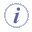When the bend deduction is read in the bend table, the K factor is not used.